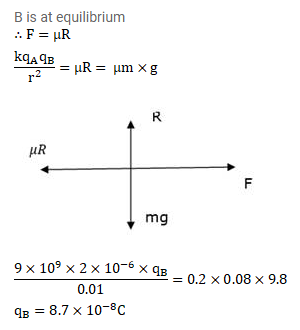# A particle A having a charge ofQuestion:

A particle A having a charge of $2.0^{\times 10^{-6}} \mathrm{C}$ is held fixed on a horizontal table. A second charged particle of mass $80 \mathrm{~g}$ stays in equilibrium on the table at a distance of $10 \mathrm{~cm}$ from the first charge. The coefficient of friction between the table and this second particle is ${ }^{\mu}=0.2$. Find the range within which the charge of this second particle may lie.

Solution: